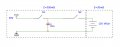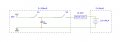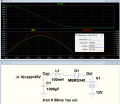# Impedance matching in capacitor discharging

#### Tutor88

Joined Feb 8, 2023
52
I have a query regarding impedance matching in the context of the discharging of a capacitor into a secondary cell.

With reference to the circuit below, a 1000uF capacitor is charged up to 30V when S1 is closed. After charging, S1 is opened and S2 is closed to discharge into a 12V Lead Acid battery.On the basis of the Power Transfer Theory, the impedance of the Source should ideally match the Load. Here, the capacitor and its connecting wires, with a total of 350mΩ (ESR of 300mΩ and 50mΩ for the wires) is not even close to that of the battery at an assumed value of 20mΩ.

My query is whether it makes a difference to the way the energy is transferred if the impedances do not match and, if it is of some importance, how can they be better matched? Perhaps one can add an adjustable choke in front of the battery so that when the discharge pulse starts, it offers a degree of inductive reactance that serves to increase the battery's overall impedance closer to that of the capacitor and connectors, the Source?

Thank you.

#### DickCappels

Joined Aug 21, 2008
9,874
You will get more current through the battery using the lowest equivalent series resistance capacitor you can find. If you figure what impedance to match, you will lose have your power unless you make a lossless matching network. A lot of trouble for no benefit in my opinion.

#### Tutor88

Joined Feb 8, 2023
52
So with a typical low ESR capacitor of the sort of value that I quoted (300mΩ) it doesn't matter that much if the total impedance is nowhere near the Load impedance of around 20mΩ? You imply that making the battery around 350mΩ would not improve the overall power transfer, so does the power transfer theory not apply here or is it that the trouble involved in matching is simply not worth the effort?

Out of interest, what does a lossless matching network involve and would my idea of an adjustable inductor not work at all?

#### Tutor88

Joined Feb 8, 2023
52
As this is a theoretical consideration for student educational purposes, and me in the process, I’m focusing on the principles rather than what is worth the effort.

I appreciate that in this context of a single DC pulse,impedance equal’s resistance as there is no reactance to speak of. I will explore the link.

Given that a transformer will have a substantial inductance I assume my idea of a choke of some form was not completely off the rails#### ericgibbs

Joined Jan 29, 2010
17,446
Hi T88,
Depending upon the battery type, the battery voltage will not hold at 12V ,it will increase and so reduce the current flow in the circuit.
E

#### BobTPH

Joined Jun 5, 2013
6,528
A battery can receive and store energy without dissipation, it is not simply an impedance.

And you are misinterpreting the max power theorem. If the source impedance can be changed, the max power will always be when you make the source impedance zero.

Let’s try some examples. Take a fixed load impedance, and a source voltage with different series resistances.

Lets’s say 10V and a a load of 10 Ohms.

With a source impedance of 10 Ohms matching the load, the current is 0.5A and power in the load is I^2 R = 2.5W

With a source impedance of 0, the current is 1A and power in the load is is 10W.

Now fix the source impedance at 10 Ohms.
With a load impedance 10 Ohms we already calculated the power as 2.5W.

With a load of 1 Ohm, the current is 10/11 = 0.91A. And the power is 0.91^2 x 1 = 0.83W.

With a load of 15 Ohms, the current is 10/25 = 0.4A and the power is 0.4^2 x 15 = 2.4W.

The max power theorem tells you the load impedance that maximizes power transfer when the source impedance is fixed.

Last edited:
•nsaspook

#### Ian0

Joined Aug 7, 2020
7,484
The lower the ESR, the higher the loss.
The power transfer theory isn’t quite what everyone thinks it is. It applies to the maximum power in the LOAD from a given source impedance, and it has nothing to do with efficiency. In fact, the efficiency at maximum power is 50%.
To transfer charge between two capacitors, the higher the resistance the lower the current and the longer it takes. The power loss is proportional to the current squared, the energy loss is the power loss x time, so the energy loss is proportional to the charge current (remember that when using a high current car charger: no only does it cost more per kWh, the losses are higher and less energy goes into the car)
So, to complete the charge transfer, the quicker it is done, the higher the loss.
To transfer power efficiently between two different voltages, you need an inductor, and the circuit is a buck or a boost regulator.

#### Tutor88

Joined Feb 8, 2023
52
But my source impedance is fixed by the ESR of the capacitor and the connecting leads. So is the internal resistance of the battery as the load. All it seems I could do would be to match them in some way for maximum energy/power transfer. Or are you saying that the optimum energy transfer is not when the two resistances are the same?

#### Tutor88

Joined Feb 8, 2023
52
I’m trying to assimilate all this into some tutoring points.

If the capacitor discharges in its normal fashion (RC time constant etc), do I get more energy to the battery by matching the resistances with a ‘matching network’ or by them remaining different? (assuming the source voltage is significantly above the load voltage)

#### Ian0

Joined Aug 7, 2020
7,484
Depends what you mean by optimum!
Do you mean that you get the most, or that you lose the least?

#### Tutor88

Joined Feb 8, 2023
52
I see what you mean but I would say get the most.

It seems that optimum energy transfer when the resistances are matched, is only 50%. So given that my source and load resistances are both fixed, would I get more of the energy from the capacitor to the battery with the resistance somehow matched (there appear to be various options on how to do that) and, if that was to occur what do I loose in terms of energy or speed etc?

#### BobTPH

Joined Jun 5, 2013
6,528
You are ignoring the battery. The load here is the battery, not the series resistance. Any power in the series resistance results in lost energy. If the idea is to transfer the most energy you can from the capacitor to the battery, you would use a buck boost converter, which can limit the current without loss to resistance.

But I will try to answer your question anyway. At any instant during the transfer, the current will be:

I = (Vcap - Vbat) / Rser

The power transferred to the battery is:

Pbat = I Vbat

The power lost is:

Plost = I (Vcap - Vbat)

So the efficiency is:

Pbat / (Pbat + Pcap) =

I Vbat / I(Vbat + Vcap - Vbat) =

I Vbat / I Vcap =

Vbat / Vcap

So the resistances do not matter at all for efficiency! Note that this is exactly the same formula as a linear voltage regulator, which makes sense because the resistors are doing just that, they are “regulating” the cap voltage down to the battery voltage.

That is why using an SMPS will allow for higher efficiency, it regulates Vcap down to be nearly the same voltage as Vbat, without the same loss.

#### Tutor88

Joined Feb 8, 2023
52
Let me try and summarise:

1. Whatever the resistances of the Source and Load, the transfer efficiency is the same. (Max power transfer theory doesn't quite work here). If so what is the value in using a capacitor with as low an ESR as possible?

2. To transfer the most energy from the capacitor to the battery, it is better to match the voltage of the load to that of the source rather than match their resistances. In this setting it would involve a boost converter (acting as a SMPS) to raise the load voltage to 30V from its 12V to match that of the source capacitor (see diagram).#### Ian0

Joined Aug 7, 2020
7,484
Assuming conventional signal flow from left to right, you will be converting 30V down to 12V to charge the battery, so you need a buck regulator.

#### ericgibbs

Joined Jan 29, 2010
17,446

#### crutschow

Joined Mar 14, 2008
32,070
I assume my idea of a choke of some form was not completely off the rails
It is not
An efficient way to transfer the energy from the capacitor to the battery is to use an inductor with a diode for resonant charging.
It's rather like a one-shot buck/boost converter.

The LTspice simulation below shows the result for a nominal 100mH inductor and a Schottky diode for maximum efficiency.
The inductor stores the capacitors energy while it is being transferred to the battery and the diode blocks any return from the battery back to the capacitor.
The capacitor voltage (green trace) goes from 30V to about -5V (due to the resonant relation between the inductor and capacitor).
The peak current (yellow trace) during the charging is a little over 1.7A.
The energy transferred (red trace) is 423mJ (small Waveform window) from the initial 450mJ stored in the capacitor (of course that doesn't include any circuit/inductor/battery resistances which would reduce that some).•cmartinez

#### BobTPH

Joined Jun 5, 2013
6,528
Simple question turned out to be not so simple, didn't it? I had to work the math to learn that the resistance does not matter, only the relative voltages of the capacitor and battery.

#### Tutor88

Joined Feb 8, 2023
52
Assuming conventional signal flow from left to right, you will be converting 30V down to 12V to charge the battery, so you need a buck regulator.
Yes of course. However, it occurs to me that the capacitor voltage will be dropping fast from 30V towards 12V over a few ms during discharge so will using a Buck converter really make that much of a difference?

Indeed